# Current of Electricity

Electric current is the rate of flow of charge. {NOT: charged particles}

Electric charge Q passing a point is defined as the product of the (steady) current at that point and the time for which the current flows,

Q = I t

One coulomb is defined as the charge flowing per second pass a point at which the current is one ampere.

Example 1:
An ion beam of singly-charged Na+ and K+ ions is passing through vacuum. If the beam current is 20 μ A, calculate the total number of ions passing any fixed point in the beam per second. (The charge on each ion is 1.6 x 10-19 C.)

Current, I = Q / t = Ne / t where N is the no. of ions and e is the charge on one ion.

No. of ions per second = N / t = I / e = (20 x 10-6) / (1.6 x 10-19) = 1.25 x 10-14

Potential difference is defined as the energy transferred from electrical energy to other forms of energy when unit charge passes through an electrical device,

V = W / Q

P. D. = Energy Transferred / Charge = Power / Current or, is the ratio of the power supplied to the device to the current flowing,

V = P / I

The volt: is defined as the potential difference between 2 pts in a circuit in which one joule of energy is converted from electrical to non-electrical energy when one coulomb passes from 1 pt to the other, ie 1 volt = One joule per coulomb

Difference between Potential and Potential Difference (PD):
The potential at a point of the circuit is due to the amount of charge present along with the energy of the charges. Thus, the potential along circuit drops from the positive terminal to negative terminal, and potential differs from points to points.

Potential Difference refers to the difference in potential between any given two points.
For example, if the potential of point A is 1 V and the potential at point B is 5 V, the PD across AB, or VAB , is 4 V. In addition, when there is no energy loss between two points of the circuit, the potential of these points is same and thus the PD across is 0 V.

Example 2:
A current of 5 mA passes through a bulb for 1 minute. The potential difference across the bulb is 4 V. Calculate:

(a) The amount of charge passing through the bulb in 1 minute.

Charge Q = I t = 5 x 10-3 x 60 = 0.3 C

(b) The work done to operate the bulb for 1 minute.

Potential difference across the bulb = W / Q
4 = W / 0.3
Work done to operate the bulb for 1 minute = 0.3 x 4 = 1.2 J

Electrical Power, P = V I = I2 / R = V2 / R

{Brightness of a lamp is determined by the power dissipated, NOT: by V, or I or R alone}

Example 3:
A high-voltage transmission line with a resistance of 0.4 Ω km-1 carries a current of 500 A. The line is at a potential of 1200 kV at the power station and carries the current to a city located 160 km from the power station. Calculate

(a) the power loss in the line.

The power loss in the line P = I2 R = 5002 x 0.4 x 160 = 16 MW

(b) the fraction of the transmitted power that is lost.

The total power transmitted = I V = 500 x 1200 x 103 = 600 MW
The fraction of power loss = 16 / 600 = 0.267

Resistance is defined as the ratio of the potential difference across a component to the current flowing through it ,

R = VI

{It is NOT defined as the gradient of a V-I graph; however for an ohmic conductor, its resistance equals the gradient of its V-I graph as this graph is a straight line which passes through the origin}

The Ohm: is the resistance of a resistor if there is a current of 1 A flowing through it when the pd across it is 1 V, ie,
1 Ω = One volt per ampere

Example 4:
In the circuit below, the voltmeter reading is 8.00 V and the ammeter reading is 2.00 A. Calculate the resistance of R.

 Resistance of R = V / I = 8 / 2 = 4.0 Ω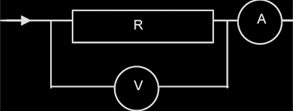#### Temperature characteristics of thermistors: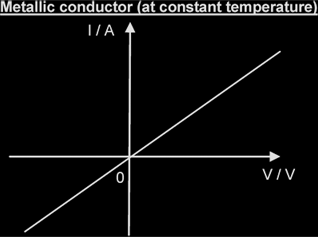The resistance (i.e. the ratio V / I) is constant because metallic conductors at constant temperature obey Ohm's Law.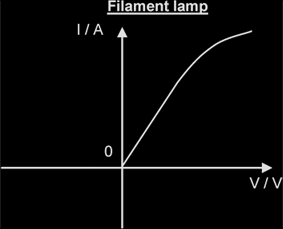As V increases, the temperature increases, resulting in an increase in the amplitude of vibration of ions and the collision frequency of electrons with the lattice ions. Hence the resistance of the filament increases with V.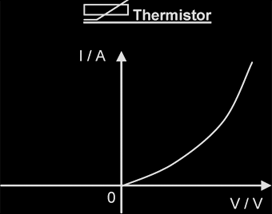A thermistor is made from semi-conductors. As V increases, temperature increases. This releases more charge carriers (electrons and holes) from the lattice, thus reducing the resistance of the thermistor. Hence, resistance decreases as temperature increases.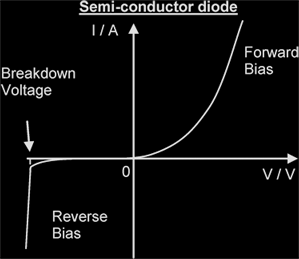In forward bias, a diode has low resistance. In reverse bias, the diode has high resistance until the breakdown voltage is reached.

Ohm's law: The current in a component is proportional to the potential difference across it provided physical conditions (eg temp) stay constant.

R = ρL / A {for a conductor of length l, uniform x-sect area A and resistivity ρ}

Resistivity is defined as the resistance of a material of unit cross-sectional area and unit length. {From R = ρl / A , ρ = RA / L}

Example 5:
Calculate the resistance of a nichrome wire of length 500 mm and diameter 1.0 mm, given that the resistivity of nichrome is
1.1 x 10-6 Ω m.

Resistance, R = ρl / A = [(1.1 x 10-6)(500 x 10-3)] / π(1 x 10-3 / 2)2 = 0.70 Ω

Electromotive force (Emf) is defined as the energy transferred / converted from non-electrical forms of energy into electrical energy when unit charge is moved round a complete circuit. ie EMF = Energy Transferred per unit charge

E = WQ

EMF refers to the electrical energy generated from non-electrical energy forms, whereas PD refers to electrical energy being changed into non-electrical energy. For example,

EMF Sources Energy Change PD across Energy Change
Chemical Cell Chem → Elec Bulb Elec → Light
Generator Mech → Elec Fan Elec → Mech
Thermocouple Thermal → Elec Door Bell Elec → Sound
Solar Cell Solar → Elec Heating element Elec → Thermal

#### Effects of the internal resistance of a source of EMF:

Internal resistance is the resistance to current flow within the power source. It reduces the potential difference (not EMF) across the terminal of the power supply when it is delivering a current.

Consider the circuit below: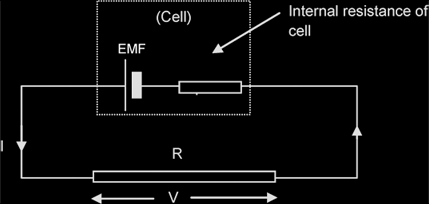The voltage across the resistor, V = IR,
The voltage lost to internal resistance = Ir
Thus, the EMF of the cell, E = IR + Ir = V + Ir
Therefore If I = 0A or if r = 0Ω, V = E